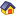Forum Home > Codejock Products > Visual C++ MFC > Chart Control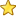New Posts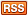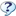FAQ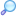Search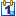Events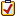Register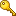Login

# pie chart gradient

 Author MessageTopic Search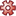Topic Options coderHNewbie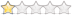Joined: 29 March 2017 Status: Offline Points: 3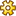Post Options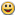Thanks(0)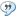Quote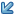Reply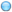Topic: pie chart gradient    Posted: 29 March 2017 at 9:52pm I have a Pie chart，as below。【question】：How to cancel the gradient of the Pie chart. I did not find “GetFillStyle()” in "CXTPChartPieSeriesStyle" class.  is there any way to cancel the gradient ？ Thank you very much.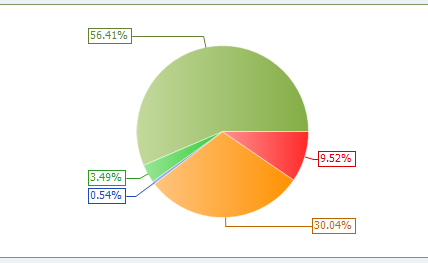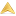coderHNewbieJoined: 29 March 2017 Status: Offline Points: 3Post OptionsThanks(0)QuoteReplyPosted: 30 March 2017 at 1:48am problem solved: GetContent()->GetAppearance()->GetPalette()->GetEntries();Modify members in Entries < placeholder="输入要翻译的句子或单词"> < value="">自动判断 < value="zh">中文< value="zh-CN">中文(简体)< value="zh-HK">中文(香港)< value="zh-TW">中文(繁体)< value="en">英语< value="ja">日语< value="ko">朝鲜语< value="de">德语< value="fr">法语< value="ru">俄语< value="th">泰语< value="af">南非语< value="ar">阿拉伯语< value="az">阿塞拜疆语< value="be">比利时语< value="bg">保加利亚语< value="ca">加泰隆语< value="cs">捷克语< value="cy">威尔士语< value="da">丹麦语< value="dv">第维埃语< value="el">希腊语< value="eo">世界语< value="es">西班牙语< value="et">爱沙尼亚语< value="eu">巴士克语< value="fa">法斯语< value="fi">芬兰语< value="fo">法罗语< value="gl">加里西亚语< value="gu">古吉拉特语< value="he">希伯来语< value="hi">印地语< value="hr">克罗地亚语< value="hu">匈牙利语< value="hy">亚美尼亚语< value="id">印度尼西亚语< value="is">冰岛语< value="it">意大利语< value="ka">格鲁吉亚语< value="kk">哈萨克语< value="kn">卡纳拉语< value="kok">孔卡尼语< value="ky">吉尔吉斯语< value="lt">立陶宛语< value="lv">拉脱维亚语< value="mi">毛利语< value="mk">马其顿语< value="mn">蒙古语< value="mr">马拉地语< value="ms">马来语< value="mt">马耳他语< value="nb">挪威语(伯克梅尔)< value="nl">荷兰语< value="ns">北梭托语< value="pa">旁遮普语< value="pl">波兰语< value="pt">葡萄牙语< value="qu">克丘亚语< value="ro">罗马尼亚语< value="sa">梵文< value="se">北萨摩斯语< value="sk">斯洛伐克语< value="sl">斯洛文尼亚语< value="sq">阿尔巴尼亚语< value="sv">瑞典语< value="sw">斯瓦希里语< value="syr">叙利亚语< value="ta">泰米尔语< value="te">泰卢固语< value="tl">塔加路语< value="tn">茨瓦纳语< value="tr">土耳其语< value="ts">宗加语< value="tt">鞑靼语< value="uk">乌克兰语< value="ur">乌都语< value="uz">乌兹别克语< value="vi">越南语< value="xh">班图语< value="zu">祖鲁语 < value="">自动选择 < value="zh">中文< value="zh-CN">中文(简体)< value="zh-HK">中文(香港)< value="zh-TW">中文(繁体)< value="en">英语< value="ja">日语< value="ko">朝鲜语< value="de">德语< value="fr">法语< value="ru">俄语< value="th">泰语< value="af">南非语< value="ar">阿拉伯语< value="az">阿塞拜疆语< value="be">比利时语< value="bg">保加利亚语< value="ca">加泰隆语< value="cs">捷克语< value="cy">威尔士语< value="da">丹麦语< value="dv">第维埃语< value="el">希腊语< value="eo">世界语< value="es">西班牙语< value="et">爱沙尼亚语< value="eu">巴士克语< value="fa">法斯语< value="fi">芬兰语< value="fo">法罗语< value="gl">加里西亚语< value="gu">古吉拉特语< value="he">希伯来语< value="hi">印地语< value="hr">克罗地亚语< value="hu">匈牙利语< value="hy">亚美尼亚语< value="id">印度尼西亚语< value="is">冰岛语< value="it">意大利语< value="ka">格鲁吉亚语< value="kk">哈萨克语< value="kn">卡纳拉语< value="kok">孔卡尼语< value="ky">吉尔吉斯语< value="lt">立陶宛语< value="lv">拉脱维亚语< value="mi">毛利语< value="mk">马其顿语< value="mn">蒙古语< value="mr">马拉地语< value="ms">马来语< value="mt">马耳他语< value="nb">挪威语(伯克梅尔)< value="nl">荷兰语< value="ns">北梭托语< value="pa">旁遮普语< value="pl">波兰语< value="pt">葡萄牙语< value="qu">克丘亚语< value="ro">罗马尼亚语< value="sa">梵文< value="se">北萨摩斯语< value="sk">斯洛伐克语< value="sl">斯洛文尼亚语< value="sq">阿尔巴尼亚语< value="sv">瑞典语< value="sw">斯瓦希里语< value="syr">叙利亚语< value="ta">泰米尔语< value="te">泰卢固语< value="tl">塔加路语< value="tn">茨瓦纳语< value="tr">土耳其语< value="ts">宗加语< value="tt">鞑靼语< value="uk">乌克兰语< value="ur">乌都语< value="uz">乌兹别克语< value="vi">越南语< value="xh">班图语< value="zu">祖鲁语 < value="YouDao">有道翻译 < value="BaiDu">百度翻译 < value="Bing">必应翻译 < value="Google">谷歌翻译 < value="GoogleCN">谷歌翻译（国内） 翻译 朗读 复制 正在查询，请稍候…… 重试 朗读 复制 复制 朗读 复制 via 译Post Reply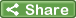Tweet

 Forum Jump -- Select Forum --  Visual C++ MFC   - Toolkit Pro   - Calendar   - Chart Control   - Command Bars   - Controls   - Docking Pane   - Property Grid   - Report Control   - Shortcut Bar   - Skin Framework   - Syntax Edit   - Task Panel   - General Discussion   - MarkupLabel Control  ActiveX COM   - Suite Pro   - Calendar   - Chart Control   - Command Bars   - Controls   - Docking Pane   - Property Grid   - Report Control   - Shortcut Bar   - Skin Framework   - Syntax Edit   - Task Panel   - General Discussion   - MarkupLabel Control  .NET WinForms   - Shortcut Bar   - Task Panel   - General Discussion  Code Snippets   - C++ Code Snippets   - Visual Basic Code Snippets  XAML Snippets   - Samples and Demo Applications  Articles and Tutorials Forum PermissionsYou cannot post new topics in this forumYou cannot reply to topics in this forumYou cannot delete your posts in this forumYou cannot edit your posts in this forumYou cannot create polls in this forumYou cannot vote in polls in this forum

This page was generated in 0.109 seconds.